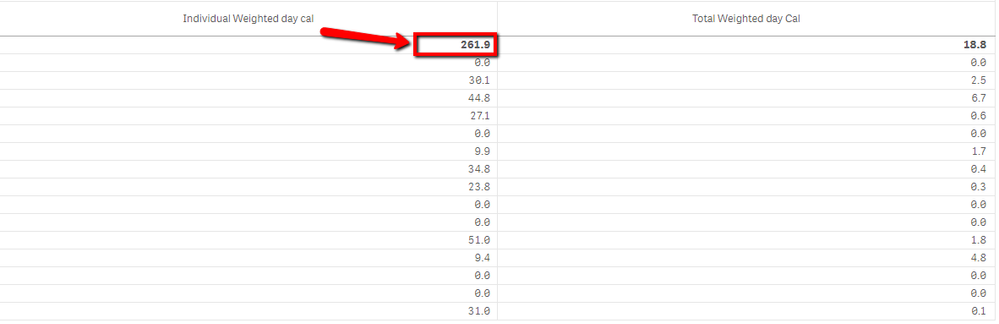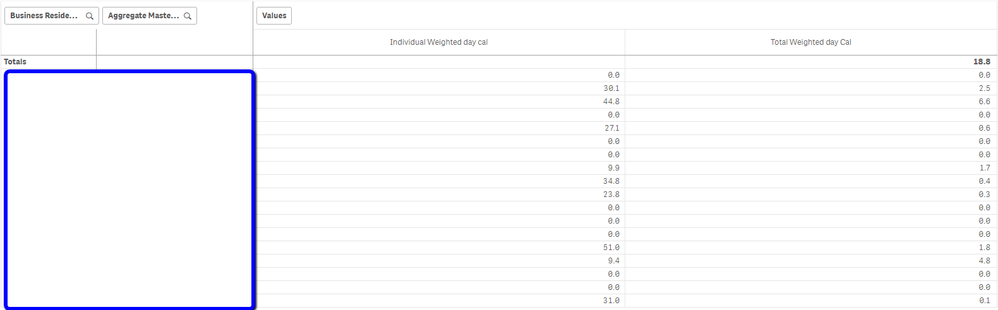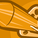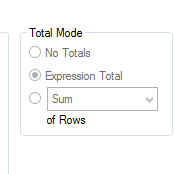# QlikView App Dev

Discussion Board for collaboration related to QlikView App Development.

Announcements
Customer & Partners, DEC. 9, 11 AM ET: Qlik Product & Strategy Roadmap Session: Data Analytics REGISTER NOW
cancel
Showing results for
Did you mean:Contributor III

## Hiding the total of a column in pivot table

Hi Everyone,

I have an issue of hiding the total of a column in the pivot table.

As highlighted below, Need to hide 261.9 value in the Pivot table.Any help is appreciated.

2 Solutions

Accepted SolutionsMVP
Hi, you can a check for dimensionality() in that expression, like:
If(Dimensionality()>0, Sum(...), '')Contributor III
Author

Hi Ruben,

This formula actually worked. Thank you so much.

If(Dimensionality()=0,
'',
(Sum(Aggr(
Sum([Days Coverge])
*
(Sum({<Calender_Year=,Calender_Year_Week=,Calender_Week_Start_Num={">=\$(vMinSelectedDate)<=\$(vMinSelectedDatePlus7Weeks)"}>}[Total Dmd])
/
Sum(total<[Aggregate Master Customer ID]>{<Calender_Year=,Calender_Year_Week=,Calender_Week_Start_Num={">=\$(vMinSelectedDate)<=\$(vMinSelectedDatePlus7Weeks)"}>}[Total Dmd]))
,[Aggregate Master Customer ID],InvSrs_MaterialID)))
)

Pivot table is displaying correctly.Thanks.

7 RepliesContributor III

select no totals for Individual weighted day cal expression in expression tab.Contributor III
Author

But I don't see anything as you mentioned in QlikSense Pivot table window.MVP
Hi, you can a check for dimensionality() in that expression, like:
If(Dimensionality()>0, Sum(...), '')Contributor III
Author

Hi Ruben,

I have 2 dimensions and 2 expressions in the pivot table.

I tried using this formula but getting Null values for the entire column. Please let me know if my expression is incorrect.

Individual weighted day =

If(dimensionality()=2,'',

(Sum(Aggr(
Sum([Days Coverge])
*
(Sum({<Calender_Year=,Calender_Year_Week=,Calender_Week_Start_Num={">=\$(vMinSelectedDate)<=\$(vMinSelectedDatePlus7Weeks)"}>}[Total Dmd])
/
Sum(total<[Aggregate Master Customer ID]>{<Calender_Year=,Calender_Year_Week=,Calender_Week_Start_Num={">=\$(vMinSelectedDate)<=\$(vMinSelectedDatePlus7Weeks)"}>}[Total Dmd]))
,[Aggregate Master Customer ID],InvSrs_MaterialID))))

ThanksMVP
Try to set a metric just with "Dimensionality()", adapt the "If(dimensionality()=2,''," to the values you will see, total rows has to lower values so maybe you need "If(dimensionality()<2,..."Contributor III
Author

I tried using the below formula as you suggested.

Individual weighted day =If(dimensionality()<2,
(Sum(Aggr(
Sum([Days Coverge])
*
(Sum({<Calender_Year=,Calender_Year_Week=,Calender_Week_Start_Num={">=\$(vMinSelectedDate)<=\$(vMinSelectedDatePlus7Weeks)"}>}[Total Dmd])
/
Sum(total<[Aggregate Master Customer ID]>{<Calender_Year=,Calender_Year_Week=,Calender_Week_Start_Num={">=\$(vMinSelectedDate)<=\$(vMinSelectedDatePlus7Weeks)"}>}[Total Dmd]))
,[Aggregate Master Customer ID],InvSrs_MaterialID))),
'')

Below is how the pivot table is displaying.Contributor III
Author

Hi Ruben,

This formula actually worked. Thank you so much.

If(Dimensionality()=0,
'',
(Sum(Aggr(
Sum([Days Coverge])
*
(Sum({<Calender_Year=,Calender_Year_Week=,Calender_Week_Start_Num={">=\$(vMinSelectedDate)<=\$(vMinSelectedDatePlus7Weeks)"}>}[Total Dmd])
/
Sum(total<[Aggregate Master Customer ID]>{<Calender_Year=,Calender_Year_Week=,Calender_Week_Start_Num={">=\$(vMinSelectedDate)<=\$(vMinSelectedDatePlus7Weeks)"}>}[Total Dmd]))
,[Aggregate Master Customer ID],InvSrs_MaterialID)))
)

Pivot table is displaying correctly.Thanks.Community Browser